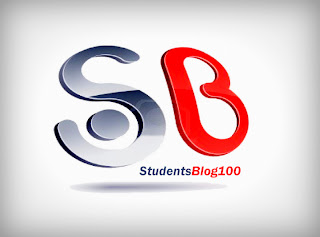IT6502 DIGITAL SIGNAL PROCESSING SYLLABUS FOR 6TH SEM CSE AND 5TH SEM IT REGULATION 2013 - Anna University Internal marks 2018
ANNA UNIVERSITY CSE SYLLABUS
IT6502 DIGITAL SIGNAL PROCESSING SYLLABUS
6TH SEM CSE SYLLABUS / 5TH SEM IT SYLLABUS
REGULATION 2013IT6502 DIGITAL SIGNAL PROCESSING SYLLABUS
OBJECTIVES:

• To introduce discrete Fourier transform and its applications.
• To teach the design of infinite and finite impulse response filters for filtering undesired signals.
• To introduce signal processing concepts in systems having more than one sampling frequency.
UNIT I SIGNALS AND SYSTEMS
Basic elements of DSP – concepts of frequency in Analog and Digital Signals – sampling theorem – Discrete – time signals, systems – Analysis of discrete time LTI systems – Z transform – Convolution – Correlation.

UNIT II FREQUENCY TRANSFORMATIONS

Introduction to DFT – Properties of DFT – Circular Convolution - Filtering methods based on DFT – FFT Algorithms - Decimation – in – time Algorithms, Decimation – in – frequency Algorithms – Use of FFT in Linear Filtering – DCT – Use and Application of DCT.

UNIT III IIR FILTER DESIGN
Structures of IIR – Analog filter design – Discrete time IIR filter from analog filter – IIR filter design by Impulse Invariance, Bilinear transformation, Approximation of derivatives – (LPF, HPF, BPF, BRF) filter design using frequency translation.

UNIT IV FIR FILTER DESIGN
Structures of FIR – Linear phase FIR filter – Fourier Series - Filter design using windowing techniques (Rectangular Window, Hamming Window, Hanning Window), Frequency sampling techniques

UNIT V FINITE WORD LENGTH EFFECTS IN DIGITAL FILTERS

Binary fixed point and floating point number representations – Comparison - Quantization noise – truncation and rounding – quantization noise power- input quantization error- coefficient quantization error – limit cycle oscillations-dead band- Overflow error-signal scaling.

TOTAL (L:45+T:15): 60 PERIODS

OUTCOMES:

Upon completion of the course, students will be able to:
• Perform frequency transforms for the signals.
• Design IIR and FIR filters.
• Finite word length effects in digital filters
TEXT BOOK:
1. John G. Proakis and Dimitris G.Manolakis, “Digital Signal Processing – Principles, Algorithms & Applications”, Fourth Edition, Pearson Education, Prentice Hall, 2007.

REFERENCES:
1. Emmanuel C.Ifeachor, and Barrie.W.Jervis, “Digital Signal Processing”, Second Edition, Pearson Education, Prentice Hall, 2002.
2. Sanjit K. Mitra, “Digital Signal Processing – A Computer Based Approach”, Third Edition, Tata Mc Graw Hill, 2007.
3. A.V.Oppenheim, R.W. Schafer and J.R. Buck, Discrete-Time Signal Processing, 8th Indian Reprint, Pearson, 2004.
4. Andreas Antoniou, “Digital Signal Processing”, Tata McGraw Hill, 2006.